Latest SSC jobs   »   SSC CGL Quantitative Aptitude : 20th...

# SSC CGL Quantitative Aptitude : 20th February 2020 for Geometry

Q1. In the given figure AM = AD, ∠B = 63° and CD is an angle bisector of ∠C, then 2∠MAC = ?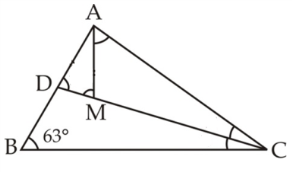(a) 54°
(b) 74°
(c) 126°
(d) 63°

Q2. The ratio between the number of sides of two regular polygons is 1 : 2 and the ratio between there interior angle is 2 : 3. The number of sides of these polygons is respectively.
(a) 6, 12
(b) 5, 10
(c) 4, 8
(d) 7, 14

Q3. Two light rods AB = a +b, CD = a –b are symmetrically parallel. The perpendicular distance between rods is a. The length of AC is given by –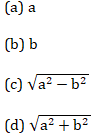Q4. One angle of pentagon is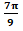. If the remaining angles are in the ratio 1 : 2 : 3 : 4, the difference between second smallest and second-largest.
(a) 150°
(b) 60°
(c) 80°
(d) 40°

Q5. In the figure given below, AB is parallel to CD. ∠ABE = 65°, ∠CDE = 15° and AB = AE. The value of ∠AEF =?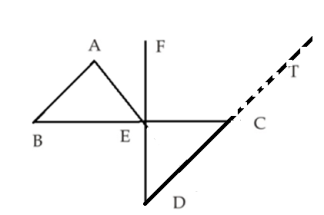(a) 30°
(b) 35°
(c) 40°
(d) 45°

#### SSC CGL 2019-20 | SSC CGL Maths Preparation | Geometry (Part 1)

Q6. In a ∆ABC, three sides are 5 cm, 4 cm and 2cm. Find the length of the median from the smallest angle vertex.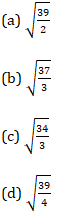Q7. In the adjoining figure, ∆ABC is an isosceles triangle, with AB = AC and ∠ABC = 50°. Then ∠BDC is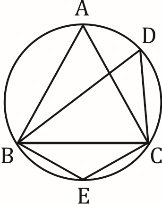(a) 110°
(b) 90°
(c) 80°
(d) 70°

Q8. In the adjoining figure A, B, C, D are the co-concyclic points. The value of ‘x’ is :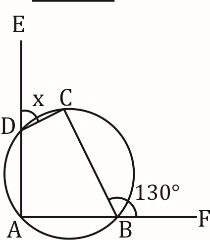lick here for Free Study Material For SSC Exams 2019-2021(a) 50°
(b) 60°
(c) 70°
(d) 90°

Q9. ∠A, ∠B, ∠C are three angles of a triangle. If ∠A -∠B =18°, ∠B – ∠C = 45°, then, ∠B, ∠A and ∠C are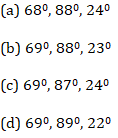Q10.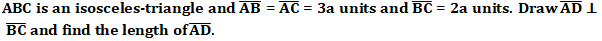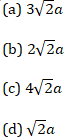#### Solutions: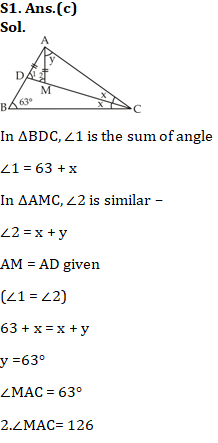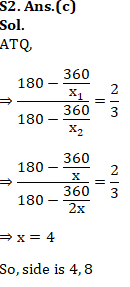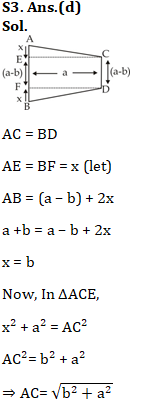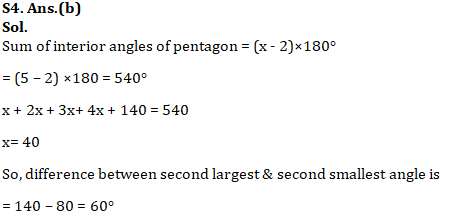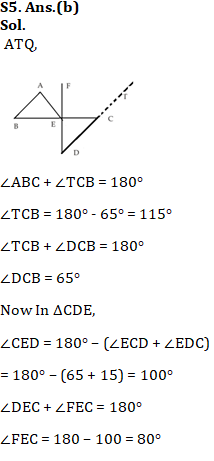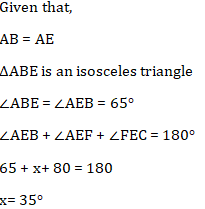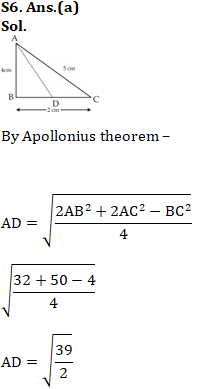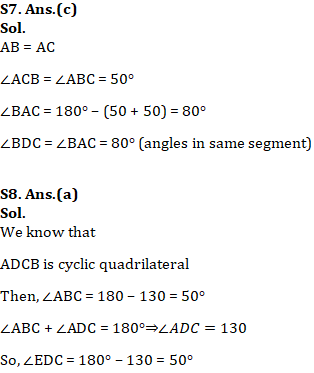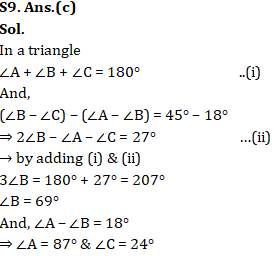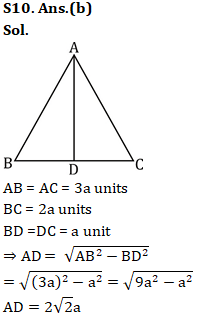#### Congratulations!General Awareness & Science Capsule PDFIncorrect details? Fill the form again here

General Awareness & Science Capsule PDF

Thank You, Your details have been submitted we will get back to you.
•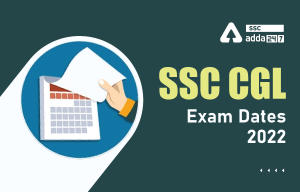SSC CGL Exam Date 2022, Check Complete E...
•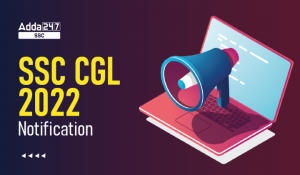SSC CGL 2022 Notification PDF Out, Apply...
•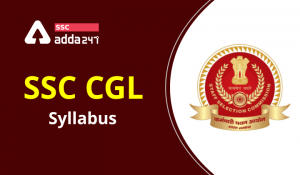SSC CGL Syllabus 2022 Out, Detailed Syll...
•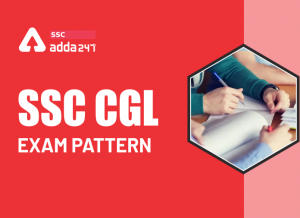SSC CGL Exam Pattern 2022, Revised Exam ...
•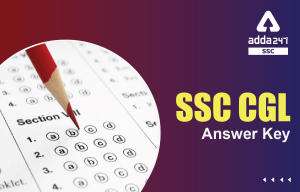SSC CGL Tier 2 Answer Key 2022 Out, Down...
•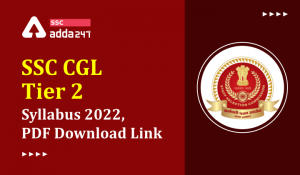SSC CGL Tier 2 Syllabus 2022, Download T...